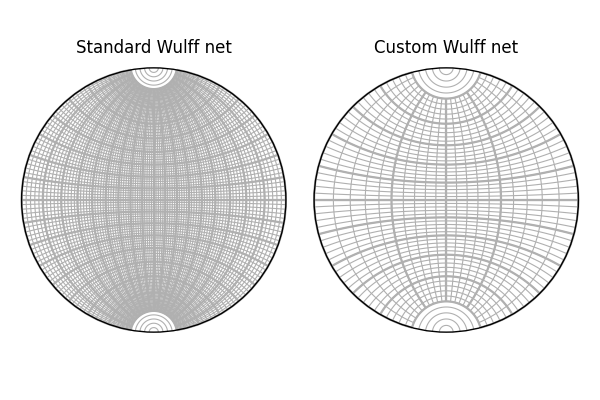# Wulff net#

This example shows how to draw a Wulff net in the stereographic projection with great and small circles.```import matplotlib.pyplot as plt
import numpy as np

from orix import plot
from orix.vector import Vector3d

n = int(90 / 2)  # Degree / net resolution
steps = 500
kwargs = dict(linewidth=0.25, color="k")

polar = np.linspace(0, 0.5 * np.pi, num=n)
v_right = Vector3d.from_polar(azimuth=np.zeros(n), polar=polar)
v_left = Vector3d.from_polar(azimuth=np.ones(n) * np.pi, polar=polar)
v010 = Vector3d.zero(shape=(n,))
v010.y = 1
v010_opposite = -v010

fig, ax = plt.subplots(figsize=(5, 5), subplot_kw=dict(projection="stereographic"))
ax.stereographic_grid(False)
ax.draw_circle(v_right, steps=steps, **kwargs)
ax.draw_circle(v_left, steps=steps, **kwargs)
ax.draw_circle(v010, opening_angle=polar, steps=steps, **kwargs)
ax.draw_circle(v010_opposite, opening_angle=polar, steps=steps, **kwargs)
for label, azimuth in zip(["B", "M''", "A", "M'"], np.array([0, 0.5, 1, 1.5]) * np.pi):
ax.text(azimuth, 0.5 * np.pi, s=label, c="C1")

fig.tight_layout()
```

Total running time of the script: ( 0 minutes 0.995 seconds)

Estimated memory usage: 9 MB

Gallery generated by Sphinx-Gallery